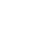# How to Calculate the Expected Return of a Stock Using the CAPM (Capitalized Asset Pricing Model)

## What is the capitalized pricing asset model?

The capitalized pricing asset model allows you to estimate your return when investing in a stock based on the level of risk that you are taking. The model assumes that a stock's price is the sum of two components: the risk-free rate, and a premium for a company-specific risk factor. This factor is typically referred to as beta or systematic risk, and it reflects how much a stock deviates from the market. If you invest in more risky stocks with higher betas, you should expect a higher stock return for that particular company.

## CAPM Formula

The formula of the capitalized pricing model looks like this:

Breaking it down, here’s what you need to know for any given stock:

1. Risk-free rate: you can easily the stock’s risk-free rate on Google by searching, “10-year treasury note for …” and using that rate.
2. Expected market return: you can also Google this value, although the historical average market return has been around 7–8%
3. Stock beta: You can calculate the stock beta manually using the formula below, or use the beta found on websites like Bloomberg and Yahoo Finance.

Once you have found all of the above values, you can calculate the stock’s estimated return using the CAPM formula.

Let’s do Apple as an example:

• The risk-free rate as of today (July 24, 2021) is 1.276%
• The expected return is 7.5%
• According to Yahoo Finance, the beta is 1.21

Altogether we find:

Apple’s expected return = 0.01276 + 1.21*(0.075–0.01276)

=0.08807 or 8.80704%

Apple’s expected return is 8.80704%. This means that if Apple did not have any specific risk factors, you could expect to make 8.80704% on your money over the course of one year

Now you know how to calculate a company’s expected return using the CAPM method.

Keep in mind this method is not perfect and has its pros and cons that you should consider. However, it is very useful to think about the relationship between the risk you are taking versus the return you should expect.

If you want to learn more about how you can get stock financials, key metrics, and growth metrics right on Excel or Google Sheets in seconds, check out www.wisesheets.io

1.graliontorile says:
1.Guillermo Valles says: